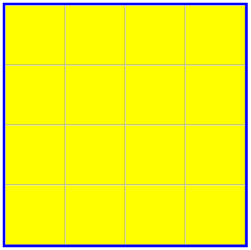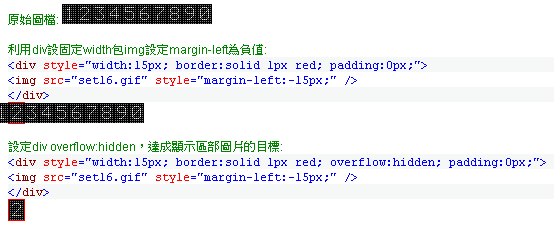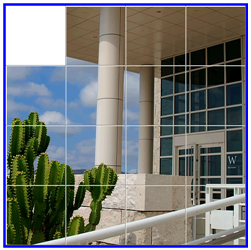# 邊做邊學 jQuery 系列 8- 益智拼圖盤

【基本排列】

``````<html xmlns="http://www.w3.org/1999/xhtml">

<meta http-equiv="Content-Type" content="text/html; charset=utf-8" />

<title>jQuery Puzzle</title>

<script type="text/javascript" src="js/jquery-1.3.1.js"></script>

<style type="text/css">

#dvPuzzle {

width: 480px; height: 480px;

border: solid 5px blue;

}

.PicCell {

width: 118px; height: 118px;

border-top: solid 1px white;

border-left: solid 1px white;

border-right: solid 1px gray;

border-bottom: solid 1px gray;

float: left;

}

</style>

<script type="text/javascript">

\$(function() {

//填入 16 張圖

for (var i=0; i<16; i++)

\$("#dvPuzzle").append("<div class='PicCell' id='Pic" + i + "'></div>");

//塗上顏色以強化展示效果

\$(".PicCell").css("background-color", "yellow");

});

</script>

<body>

<div id="dvPuzzle"></div>

</body>

</html>
``````【加上圖檔】``````\$(function() {

//填入 16 張圖

for (var i=0; i<16; i++)

{

\$("#dvPuzzle").append("<div class='PicCell' id='Pic" + i + "'><img src='Building.jpg' /></div>");

var row = parseInt(i / 4);

var col = i % 4;

\$("#Pic" + i + " img").css("margin-left", col * -120 + 1).css("margin-top", row * -120 + 1);

}

//將左上角圖塊移除

\$("#Pic0 img").remove();

});
``````【演算邏輯】

``````\$(function() {

//將位置轉成座標的換算表

var posConv = { };

//填入 16 張圖

for (var i=0; i<16; i++)

{

\$("#dvPuzzle").append("<div class='PicCell' id='Pic" + i + "'><img src='Building.jpg' /></div>");

var row = parseInt(i / 4);

var col = i % 4;

\$("#Pic" + i + " img").css("margin-left", col * -120 + 1).css("margin-top", row * -120 + 1);

//第 i 個換成第 row 列第 col 行

posConv[i] = { row:row, col:col };

}

//將左上角圖塊移除

\$("#Pic0 img").remove();

//取得四周相鄰的位置

function getNearPos(i) {

var pool = [];

var row = posConv[i].row, col = posConv[i].col;

//toCheck 用來放入待比對的對象

if (row > 0) //上

pool.push((row - 1) *  4 + col);

if (row < 4) //下

pool.push((row + 1) * 4 + col);

if (col  >  0) //左

pool.push( i - 1);

if (col < 4) //右

pool.push(i + 1);

return pool;

}

});
``````

``````//點選動作

\$(".PicCell").click(function() {

//找尋上下左右有沒有 Pic0，有則可以與它交換位置

//先找出元素是 16 個中第幾個?

var cells = \$("#dvPuzzle div");

var i = cells.index(this);

var toCheck = getNearPos(i);

while (toCheck.length > 0) {

var j = toCheck.pop();

if (cells.eq(j).attr("id") == "Pic0") //為空白格，交換位子

{

//排序，必要時對調，讓 i < j

if (i > j) { var k = j; j = i; i = k; }

var behind = cells.eq(j);

var behindPrev = behind.prev();

//左右對調

if (Math.abs(i - j) == 1)

else //上下對調

{

}

break;

}

}

});
``````

``````\$("input:button").click(function() {

for (var i = 0; i < 500; i++) {

var cells = \$("#dvPuzzle div");

//找出空格所在位置，並取得其相鄰圖塊

var toMove = getNearPos(cells.index(\$("#Pic0")));

cells.eq(toMove[ //由空格的相鄰圖塊擇一挪動

parseInt(Math.random() * toMove.length)

]).click();

}

});
``````【範例檔案下載】回到首頁